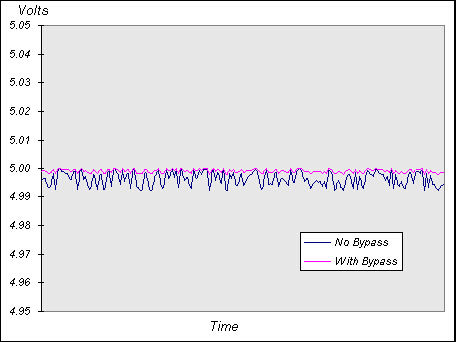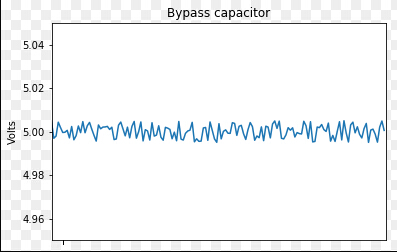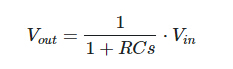## Is a laplace transform on simple low pass filter in python useful to solve my problem?

Typically: "How do I... ", "How can I... " questions
Asaki
Posts: 2
Joined: 30 Mar 2017, 10:30

### Is a laplace transform on simple low pass filter in python useful to solve my problem?

Looking at this pictureIt shows a simple graph of an with and without bypass filter circuit voltage difference, I wanted to recreate this picture.

I have coded the noise and created the graph of the noisy signal:

Code: Select all

``````import numpy as np
import matplotlib.pyplot as plt
%matplotlib inline

v_in = np.random.uniform(-0.005,0.005,150)+5
v_out = 1/(1+1*0.1E-6*s)*v_in #this line is wrong, no such thing as '*s'

plt.plot(v_in)
plt.plot(v_out)
plt.axis([0, 150, 4.95, 5.05])
plt.title('Bypass capacitor')
plt.ylabel('Volts')
plt.xticks(v_in, " ")
plt.show()``````Problem

Now the difficult part is that I want to solve this by having the parallel capacitor be represented as an RC low pass circuit with R = 1, and the corosponding transfer function ofI am confused with what to do with the 's', I think a laplace transform of the input? please help, i can work with impedances and AC-frequency, but a complex signal is new. a bit of theory behind the laplace 's' variable followed by the simple demo partialy set up would be very much appriciated!